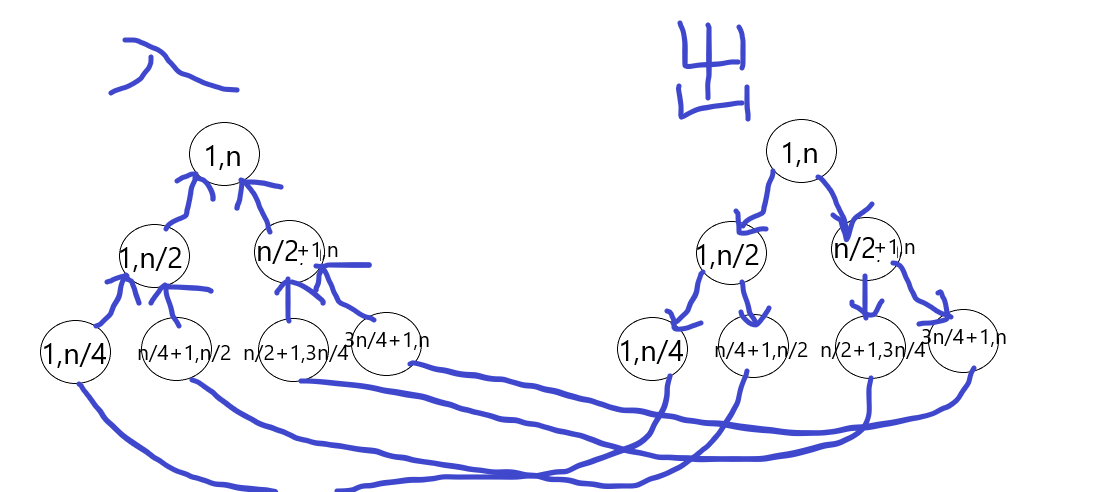# Legacy CodeForces - 787D （线段树优化建图+最短路）

## Legacy

Rick and his co-workers have made a new radioactive formula and a lot of bad guys are after them. So Rick wants to give his legacy to Morty before bad guys catch them.

There are n planets in their universe numbered from 1 to n. Rick is in planet number s (the earth) and he doesn't know where Morty is. As we all know, Rick owns a portal gun. With this gun he can open one-way portal from a planet he is in to any other planet (including that planet). But there are limits on this gun because he's still using its free trial.By default he can not open any portal by this gun. There are q plans in the website that sells these guns. Every time you purchase a plan you can only use it once but you can purchase it again if you want to use it more.

Plans on the website have three types:

1. With a plan of this type you can open a portal from planet v to planet u.
2. With a plan of this type you can open a portal from planet v to any planet with index in range [l, r].
3. With a plan of this type you can open a portal from any planet with index in range [l, r] to planet v.

Rick doesn't known where Morty is, but Unity is going to inform him and he wants to be prepared for when he finds and start his journey immediately. So for each planet (including earth itself) he wants to know the minimum amount of money he needs to get from earth to that planet.

Input

The first line of input contains three integers nq and s (1 ≤ n, q ≤ 105, 1 ≤ s ≤ n) — number of planets, number of plans and index of earth respectively.

The next q lines contain the plans. Each line starts with a number t, type of that plan (1 ≤ t ≤ 3). If t = 1 then it is followed by three integers vu and w where wis the cost of that plan (1 ≤ v, u ≤ n, 1 ≤ w ≤ 109). Otherwise it is followed by four integers vlr and w where w is the cost of that plan (1 ≤ v ≤ n, 1 ≤ l ≤ r ≤ n, 1 ≤ w ≤ 109).

Output

In the first and only line of output print n integers separated by spaces. i-th of them should be minimum money to get from earth to i-th planet, or  - 1 if it's impossible to get to that planet.

Examples

Input

3 5 1
2 3 2 3 17
2 3 2 2 16
2 2 2 3 3
3 3 1 1 12
1 3 3 17


Output

0 28 12


Input

4 3 1
3 4 1 3 12
2 2 3 4 10
1 2 4 16


Output

0 -1 -1 12


Note

In the first sample testcase, Rick can purchase 4th plan once and then 2nd plan in order to get to get to planet number 2.

q条路径有可能有这么几种形式：AC代码：

#include<cstdio>
#include<iostream>
#include<queue>
#define md(l,r) (((l)+(r))>>1)
#define rson(x) ((x)<<1|1)
#define lson(x) ((x)<<1)
typedef int Int;
#define int long long
using namespace std;
typedef long long LL;
const int size=1e5+5;
const int maxn=1e6+5;
const int inf=1e18+5;
struct node{
int l,r;
int id;
};
struct Edge{
int u,v,w;
Edge(int u=0,int v=0,int w=0):u(u),v(v),w(w){}
};
struct roar{
int id,w;
roar(int id=0,int w=0):id(id),w(w){}
friend bool operator<(roar a,roar b)
{
return a.w>b.w;
}
};
priority_queue<roar> q;
vector<Edge> edge[maxn];
int dis[maxn],vis[maxn];
int cnt=0;
void AddEdge(int u,int v,int w)
{
edge[u].push_back(Edge(u,v,w));
}
int build(int k,int l,int r,node tree[],int *no,bool type)
{
tree[k].l=l,tree[k].r=r;tree[k].id=cnt++;
if(l==r)
{
return no[l]=tree[k].id;
}
int mid=md(l,r);
if(type)//从上向下建边
AddEdge(tree[k].id,build(lson(k),l,mid,tree,no,type),0),
AddEdge(tree[k].id,build(rson(k),mid+1,r,tree,no,type),0);
else//从下向上建边
AddEdge(build(lson(k),l,mid,tree,no,type),tree[k].id,0),
AddEdge(build(rson(k),mid+1,r,tree,no,type),tree[k].id,0);
return tree[k].id;
}
void query(int k,int l,int r,int faSno,int w,node tree[],bool type)
{
if(tree[k].l>=l&&tree[k].r<=r)
{
if(type) AddEdge(faSno,tree[k].id,w);
else AddEdge(tree[k].id,faSno,w);
return ;
}
int mid=md(tree[k].l,tree[k].r);
if(r<=mid) query(lson(k),l,r,faSno,w,tree,type);
else if(l>=mid+1) query(rson(k),l,r,faSno,w,tree,type);
else query(lson(k),l,mid,faSno,w,tree,type),query(rson(k),mid+1,r,faSno,w,tree,type);
}
void Dijkstra(int beg)
{
fill(dis,dis+maxn,inf);
fill(vis,vis+maxn,inf);
while(!q.empty()) q.pop();
q.push(roar(beg,0)),dis[beg]=0;
while(!q.empty())
{
roar s=q.top();
q.pop();
int id=s.id;
if(dis[id]!=s.w) continue;
for(int i=0;i<edge[id].size();i++)
{
if(dis[edge[id][i].v]>dis[id]+edge[id][i].w)
{
dis[edge[id][i].v]=dis[id]+edge[id][i].w;
q.push(roar(edge[id][i].v,dis[edge[id][i].v]));
}
}
}
}
node intree[size<<2],outtree[size<<2];
int inno[size],outno[size];
Int main()
{
int n,q,s;
scanf("%lld%lld%lld",&n,&q,&s);
build(1,1,n,intree,inno,0);
build(1,1,n,outtree,outno,1);
for(int i=1;i<=n;i++) AddEdge(inno[i],outno[i],0),AddEdge(outno[i],inno[i],0);
for(int i=1;i<=q;i++)
{
int op;
scanf("%lld",&op);
if(op==1)
{
int u,v,w;
scanf("%lld%lld%lld",&u,&v,&w);
AddEdge(inno[u],outno[v],w);
}
else if(op==2)
{
int v,l,r,w;
scanf("%lld%lld%lld%lld",&v,&l,&r,&w);
query(1,l,r,inno[v],w,outtree,1);
}
else
{
int v,l,r,w;
scanf("%lld%lld%lld%lld",&v,&l,&r,&w);
query(1,l,r,outno[v],w,intree,0);
}
}
Dijkstra(inno[s]);
for(int i=1;i<=n;i++)
{
if(dis[outno[i]]!=inf)printf("%lld ",dis[outno[i]]);
else printf("-1 ");
}
cout<<endl;
}